### Home > CALC > Chapter 7 > Lesson 7.3.2 > Problem7-120

7-120.
1. Find the antiderivative of the following differential equations. Use implicit integration when necessary. Solve your equations for y. Be careful about introducing the constant of integration at the appropriate time. Homework Help ✎

1.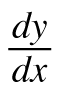= 7x + 3

2.= 7y + 3

3.= 7y2

4.= 7

5.= ey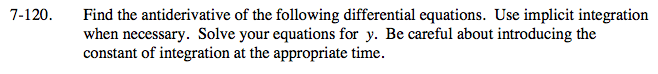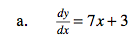Notice that the derivative is in terms of x. Antidifferentiate!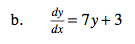Compare and contrast part (a) with part (b).
Which one requires implicit integration?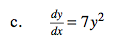Translation: The derivative is equal the the original function squared times a constant.
What can you conclude about the original function?
Is it linear, quadratic, cubic, exponential, trigonometric...?
Use implicit integration to check your conjecture.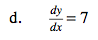Translation: The derivative of this function is a constant.
What can you conclude about the function?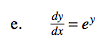y = −ln|−x + C|
Explain the significance of the absolute value.